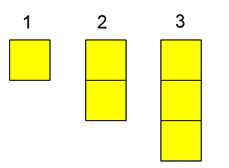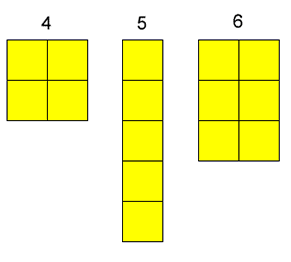# Numbers as Shapes

## Numbers as Shapes

Here are the numbers $1$ to $6$ drawn using coloured squares:We can call these numbers squares, rectangles or sticks.

$1$ is a square but just a single one.
We can't make $2$ into a square so $2$ is a stick.
We can't make $3 £ into a square or a rectangle so$3% can only be a stick.
We can make $4$ into a square.
We can't make $5$ into a square or a rectangle, so $5$ can only be a stick.
We can't make $6$ into a square, but we can make $6$ into a rectangle.

If you had $7$ yellow squares, what could you make them into? A square, a rectangle or a stick? How about $8$ squares?

Try this with the numbers up to and including $20$.

Which ones can only be sticks?
Which ones can make rectangles?
Which ones are squares?

What do you notice?

This problem was inspired by a session at an ATM conference, led by Tandi Clausen-May.

### Why do this problem?

The idea of this problem is to give pupils a tool for visualising numbers.  It is not intended to teach the vocabulary of square numbers, multiples and primes necessarily.

### Possible approach

You could show the group the picture of the numbers  $1$ to $6$, or show them models made from interlocking cubes.  Alternatively, you could use the interactive teaching program called Area to create the numbers by colouring squares.  Whichever medium you use, simply ask the children to chat to someone else about what they notice.  After giving them a few moments, draw them together as a whole group to discuss their thoughts.  Use the children's ideas to introduce the 'square', 'stick' and 'rectangle' classification and then set them off investigating for numbers $7$ to $20$ inclusive (and beyond).  It may come up in conversation that the 'sticks' are also rectangles so children may find their own ways of naming the three categories.

It would be very valuable for pupils to be able to physically make the numbers with bricks, interlocking cubes, square tiles or by colouring squared paper.

After a suitable period of time, bring everyone back together to discuss their findings.  At this point, some children could show models or drawings or the interactive program.  Can the whole class group the numbers?  Invite the children to comment on anything they notice.  Listen out for those who mention, for example, odd/even numbers.  Can anyone explain why some of the numbers can only be made into sticks?  This might be a good opportunity to introduce specific vocabulary.

### Key questions

Tell me what you've tried so far.
How are you going to record what you've done?

### Possible extension

Trying higher numbers will appeal to some children.  Encourage them to predict what 'kind' of number each will be before creating a model or drawing. Some children will enjoy learning the words to describe these numbers - squares, multiples and primes.

### Possible support

It would be a good idea for learners to have some cubes or tiles to make the numbers with, or to use some squared paper and colour the squares.